Learning Library
Who we are
Guidance

# 5.NF.B.6 Worksheets, Workbooks, Lesson Plans, and Games

#### CCSS.MATH.CONTENT.5.NF.B.6

:
"Solve real world problems involving multiplication of fractions and mixed numbers, e.g., by using visual fraction models or equations to represent the problem."

These worksheets and lesson plans can help students practice this Common Core State Standards skill.

## Worksheets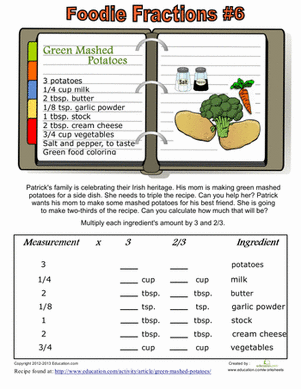Recipe Fractions #6
Worksheet
Recipe Fractions #6
Fraction practice can be fun when you add food into the mix! Your child will multiply fractions to change the amounts of ingredients in a recipe.
Math
Worksheet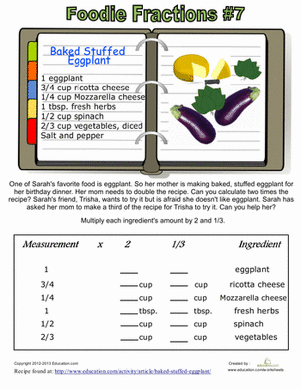Recipe Fractions #7
Worksheet
Recipe Fractions #7
Practice multiplying fractions using a real recipe. Your child will have a chance to learn about food as she learns about math.
Math
Worksheet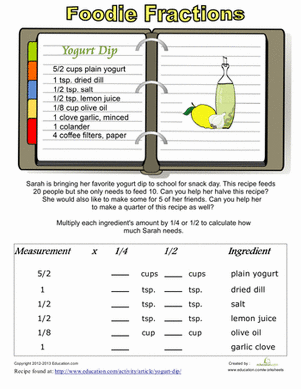Recipe Conversion
Worksheet
Recipe Conversion
Introduce your little math champ to fraction multiplication in the kitchen! He'll get a taste of measurements using a recipe to calculate the fractions.
Math
Worksheet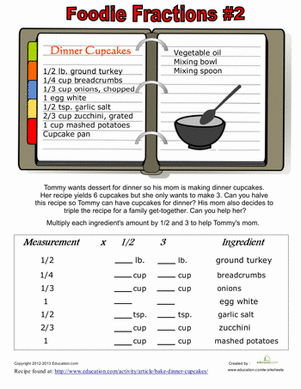Recipe Fractions #2
Worksheet
Recipe Fractions #2
Use food and math together to learn fractions! Help your child multiply fractions to convert these recipes to smaller or larger portions.
Math
Worksheet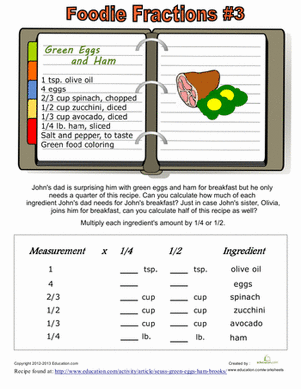Recipe Fractions #3
Worksheet
Recipe Fractions #3
Combine math with the fun of cooking! Practice fraction multiplication using the measurements of ingredients from a recipe.
Math
Worksheet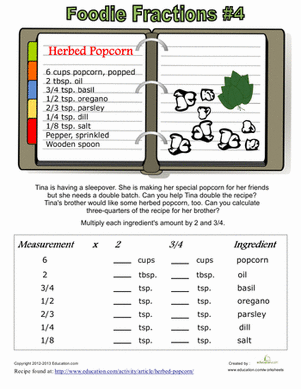Recipe Fractions #4
Worksheet
Recipe Fractions #4
Practice multiplying fractions while learning to cook in the kitchen! Your little mathematical chef will adjust the measurements of a real recipe.
Math
Worksheet

## Lesson PlansMixed Word Problems with RDW Strategy
Lesson plan
Mixed Word Problems with RDW Strategy
This end-of-the-school-year lesson will get the students focused on all the things they learned in math class! It reviews different skills with fractions and decimals in word problems using the "Read, Draw, and Write" strategy.
Math
Lesson plan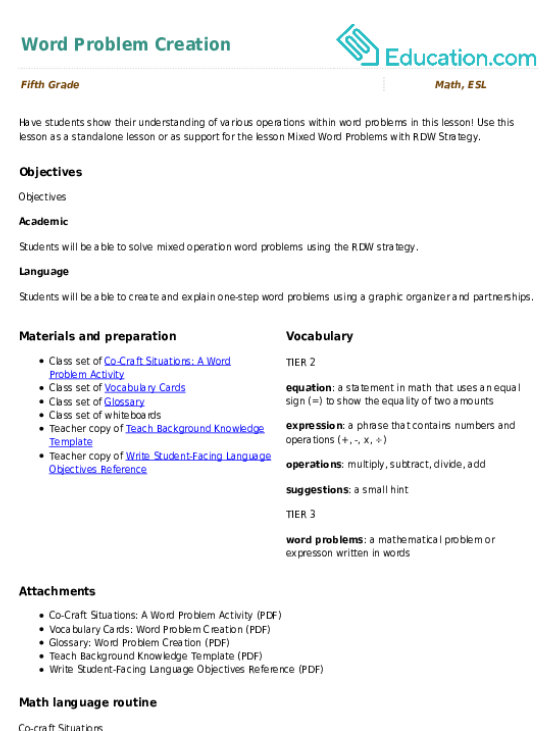Word Problem Creation
Lesson plan
Word Problem Creation
Have students show their understanding of various operations within word problems in this lesson! Use this lesson as a standalone lesson or as support for the lesson Mixed Word Problems with RDW Strategy.
Math
Lesson plan

## Workbooks

No workbooks found for this common core node.

## Games

No games found for this common core node.

## Exercises

No exercises found for this common core node.

What could we do to improve Education.com?

Please note: Use the Contact Us link at the bottom of our website for account-specific questions or issues.

What would make you love Education.com?